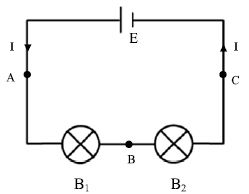# What is Series Circuit?

The circuit in which the electric components are connected one after another in a single loop is called a series circuit. A series circuit is a closed circuit in which the current follows one path, as opposed to a parallel circuit where the circuit is divided into two or more paths. In a series circuit, the current through each load is the same and the total voltage across the circuit is the sum of the voltages across each load.

By arranging a cell E, two bulbs B1 and E1 one after another a series circuit is formed in figure. As there is a single path in the circuit, the same current will flow throughout the whole circuit. Now if the ammeter is connected at points A, B or C, the value of the electric current will found to be the same.The little bulbs that are used for decoration purpose in wedding ceremony or in different programs are connected in series. We increase the voltage by connecting more than one battery in series in a torch light. The ammeter is connected in series to measure the electric current in a circuit.